Function Repository Resource:

# TangentialAngle

Compute the tangential angle of a curve

Contributed by: Wolfram Staff (original content by Alfred Gray)
 ResourceFunction["TangentialAngle"][c,t] computes the tangential angle of the curve c parameterized by variable t.

## Details and Options

The tangential angle φ of a plane curve is defined as ρ dφ=ds, where ρ is the radius of curvature and s the arc length. Integration gives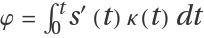, where κ=1/ρ.
Gray (1997) calls it the turning angle.

## Examples

### Basic Examples (4)

Tangential angle for a curve:

 In:=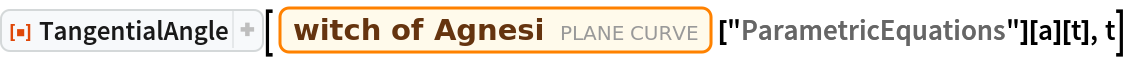Out=Tangential angle for several curves:

 In:=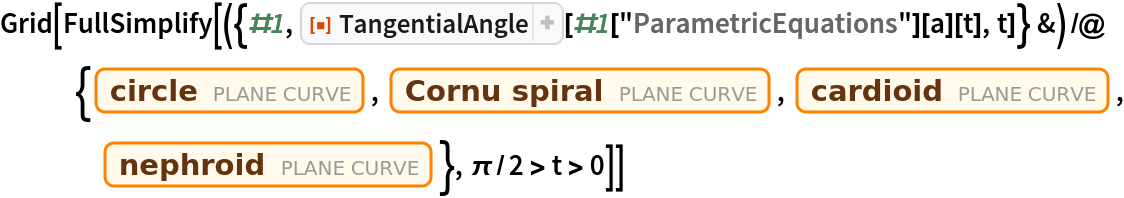Out=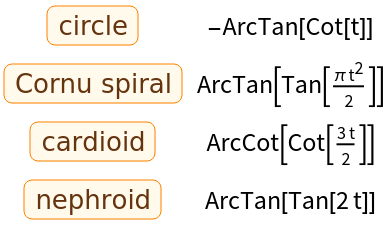A more complicated result:

 In:=Out=The ellipse pedal curve:

 In:=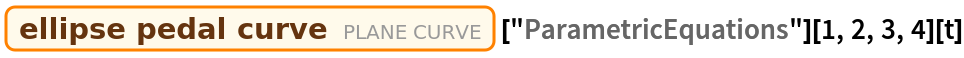Out=Plot the curve:

 In:=Out=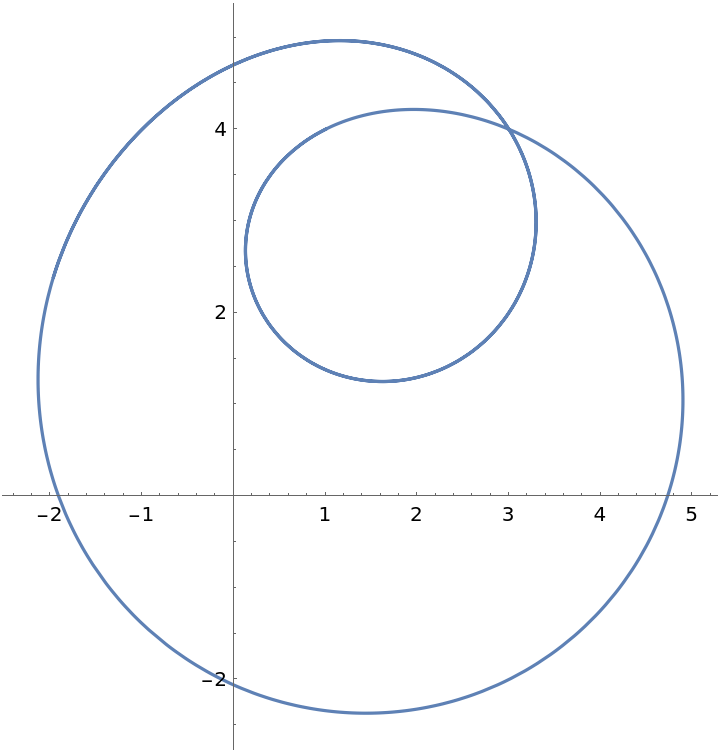The tangential angle:

 In:=Out=### Properties and Relations (2)

For some named curves, it is possible to get the tangential angle using entities, but the output can be written in a different way:

 In:=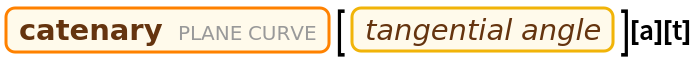Out=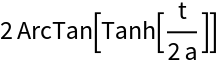In:=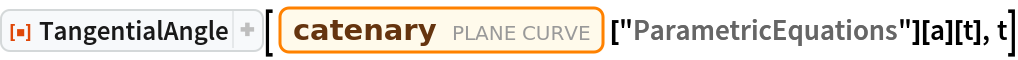Out=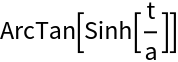Evaluating at one point:

 In:=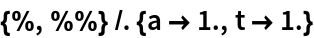Out=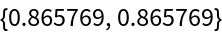### Possible Issues (1)

Some cases may need some time to compute:

 In:=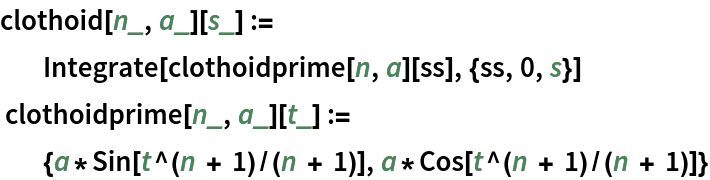In:=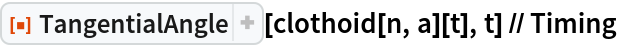Out=Enrique Zeleny

## Version History

• 1.0.0 – 30 September 2020